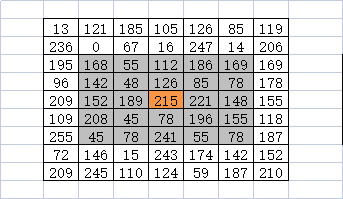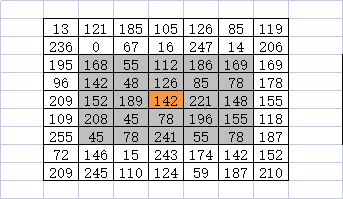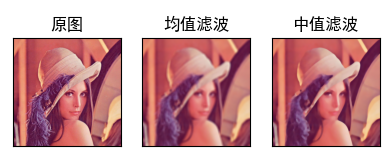﻿ opencv 中值滤波 Median filtering – 蒋智昊的博客# opencv 中值滤波 Median filtering```import cv2
import matplotlib.pylab as plt
import matplotlib as mpl

mpl.rcParams['font.sans-serif'] = ['SimHei']  # 指定默认字体为黑体
mpl.rcParams['axes.unicode_minus'] = False    # 正常显示负号

# 均值滤波

# 解决彩色图像出现色差问题
b, g, r = cv2.split(img)  # 分别提取B、G、R通道
img_new = cv2.merge([r, g, b])  # 重新组合为R、G、B

blur = cv2.blur(img_new, (25, 25))

blur_med = cv2.medianBlur(img_new, 25)

blur_gauss = cv2.blur(img_new, (25, 25), 0)

# 显示图像
# plt.subplot(nrows, ncols, index)
# 表示在当前画布的右上角创建一个1行3列的绘图区域，同时 选择在第 1 个位置绘制子图。
plt.subplot(1, 4, 1)
plt.xticks([]), plt.yticks([])  # 隐藏x和y轴
plt.imshow(img_new)
plt.title('原图')

plt.subplot(1, 4, 2)
plt.xticks([]), plt.yticks([])  # 隐藏x和y轴
# plt.imshow(blur, 'gray')
plt.imshow(blur)
plt.title('均值滤波')

plt.subplot(1, 4, 3)
plt.xticks([]), plt.yticks([])  # 隐藏x和y轴
plt.imshow(blur_med)
plt.title('中值滤波')

plt.subplot(1, 4, 4)
plt.xticks([]), plt.yticks([])  # 隐藏x和y轴
plt.imshow(blur_gauss)
plt.title('高斯滤波')
plt.show()
```